Click to Chat

1800-1023-196

+91-120-4616500

CART 0

• 0

MY CART (5)

Use Coupon: CART20 and get 20% off on all online Study Material

ITEM
DETAILS
MRP
DISCOUNT
FINAL PRICE
Total Price: Rs.

There are no items in this cart.
Continue Shopping• Complete Physics Course - Class 11
• OFFERED PRICE: Rs. 2,968
• View Details

```Solved Problems on Conservation of Momentum:-

Problem 1:-

A 4.88-kg object with a speed of 3.14 m/s strikes a steel plate at an angle of 42.0º and rebounds at the same speed and angle as shown in the below figure. What is the change (magnitude and direction) of the linear momentum of the object?

Concept:-

Momentum of the object (p) is defined as the mass of the object (m) time’s velocity of the object (v).

p = mv      …… (1)

Solution:-

The below figure shows a 4.88-kg object with a speed of 31.4 m/s strikes a steel plate at an angle of 42.0° and rebounds at the same speed with same angle which is shown geometrically.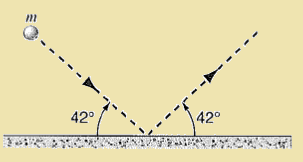The initial momentum of the object is pi when it strikes the steel plate.

To find initial momentum pi, substitute pi for p, 4.88-kg for mass m of the object and 31.4 m/s for velocity of the object in the equation p = mv,

pi = mv

= (4.88 kg) (31.4 m/s)

= 153 kg. m/s        …… (2)

Since the final momentum pf of the object is equal to the initial momentum pi of the object, therefore,

pf = 153 kg. m/s      …… (3)

In the above figure the angle θ will be,

θ = 42° + 42°

= 84°

Using cosine law, the magnitude of change of the linear momentum of the object Δp will be,

Δp = √( pi)2 + (pf)2 + 2 (-pi)(pf)cos θ     …… (4)

To obtain magnitude of linear momentum Δp, substitute 153 kg. m/s for pi, 153 kg. m/s for pf and 84° for the angle θ in the equation Δp = √( pi)2 + (pf)2 + 2 (-pi)(pf) cos θ,

Δp = √( pi)2 + (pf)2 + 2 (-pi)(pf) cos θ

= √(153 kg. m/s)2 + (153 kg. m/s)2 - 2 (153 kg. m/s)( 153 kg. m/s) cos 84°

= 205 kg. m/s

From the above observation we conclude that, the change of the linear momentum of the object will be, 205 kg. m/s and also from the figure the direction of change of the linear momentum of the object is perpendicular to the steel plate.

____________________________________________________________

Problem 2:-

Below figure shows an approximate representation of force versus time during the collision of a 58-g tennis ball with a wall. The initial velocity of the ball is 32 m/s perpendicular to the wall; it rebounds with the same speed, also perpenducular to the wall. What is the value of Fmax, the maximum contact force during the collision?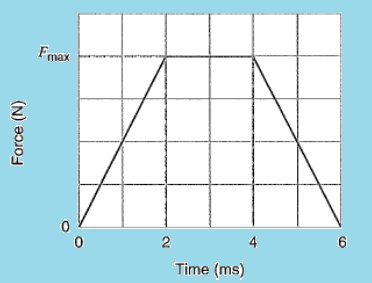Concept:-

Momentum of a body (p) is defined as,

p = mv

Here, m is the mass of the body and v is the velocity of the body.

The impulse of the net force J acting on a particle during a given time interval is equal to the change in momentum Δp of the particle during that interval.

So, J = Δp

= pf - pi

Here pf is the final momentum and pi is the initial momentum of the body.

Again the impulse is the area under a force-time graph.

Solution:-

As the tennis ball strikes with the wall having velocity v and then rebounds with same velocity, thus the change in momentum Δp of the tennis ball will be equal to,

Δp = (mv) – (-mv)

= 2mv

To obtain the change in momentum Δp of the ball, substitute 58-g for m and 32 m/s for v in the equation Δp = 2mv, we get,

Δp = 2mv

= 2 (58-g) (32 m/s)

=  2 (58-g×10-3 kg/1 g) (32 m/s)

= 3.7 kg.m/s

To obtain the impulse we have to find out the area under force-time graph for the trapezoid.

So, J = Fmax (2 ms+6 ms)/2

= Fmax (4 ms)

So, Fmax = J/4 ms

= Δp/4 ms

To obtain the maximum contact force Fmax during the collision, substitute 3.7 kg.m/s for Δp in the equation Fmax= Δp/4 ms,

Fmax= Δp/4 ms

= (3.7 kg.m/s) / (4 ms)

= (3.7 kg.m/s) / (4 ms×10-3 s/1 ms)

= 930 kg. m/s2

= (930 kg. m/s2) (1 N/1 kg. m/s2)

= 930 N

From the above observation we conclude that, the maximum contact force Fmax during the collision would be 930 N.

_______________________________________________________________________

Problem 3:-

A croquet ball with a mass 0.50 kg is struck by a mallet, receiving the impulse shown in the graph below. What is the ball’s velocity just after the force has become zero?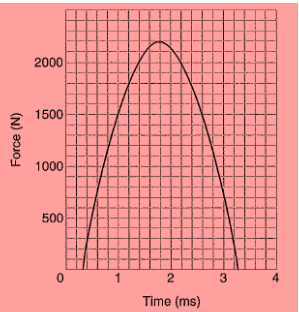Concept:-

The impulse of the net force J acting on a particle during a given time interval is equal to the change in momentum Δp of the particle during that interval.

Jx = Δp

= pfx – pix

Here pfx is the final momentum and pix is the initial momentum of the body.

So, pfx = Jx + pix

Momentum px of a particle is equal to the mass of the particle times velocity vx of the particle.

So, px = m vx

Or, vx = px/m

Solution:-

To obtain the area, we have to use Simpson’s rule.

Using Simpson’s rule, the area will be,

Jx = 1/3 h (f0 +4f1+2f2+4f3+……+4f13 + f14)

Here h (=0.2 ms) is the width of each strip.

So, Jx = 1/3 h (f0 +4f1+2f2+4f3+……+4f13 + f14)

= 1/3 (0.2 ms) (200+4(800)+2(1200))N

=  1/3 (0.2 ms×10-3 s/1 ms) (200+4(800)+2(1200))N

= (4.28 N.s) (1 kg.m/s2/ 1 N)

= 4.28 kg.m/s

The impulse is the change in momentum and the ball started from rest. So the initial momentum of the ball will be zero (pix=0).

To obtain the final momentum pfx, substitute 4.28 kg.m/s for Jx and 0 m/s for pix in the equation pfx = Jx + pix,

pfx = Jx + pix

= 4.28 kg.m/s + 0 m/s = 4.28 kg.m/s

To obtain the final velocity vfx, substitute 4.28 kg.m/s  for  pfx and 0.50 kg for m in the equation vfx = pfx/m ,

vfx = pfx

= (4.28 kg.m/s) / (0.5 kg)

= 8.6 m/s

From the above observation we conclude that, the final velocity  vfx of the ball will be 8.6 m/s.

________________________________________________________________

Problem 4:-

Meteor Crater in Arizona as shown in the below figure is thought to have been formed by the impact of a meteorite with the Earth some 20,000 years ago. The mass of the meteorite is estimated to be 5×1010 kg and its speed to have been 7.2 km/s. What speed would such a meteorite impart to the Earth in a head-on collision?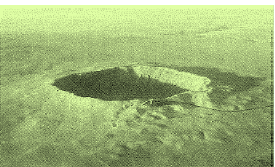Concept:-

Momentum of the body p is equal to the mass of the body m times velocity of the body v.

So, p = mv

In accordance to the principle of conservation of energy, the final momentum of the system is equal to the initial momentum of the system.

Consider the initial momentum of the Earth is pi,1, initial momentum of the meteorite is pi,2, final momentum of the Earth is pf,1 and final momentum of the meteorite is pf,2.

To obtain the initial momentum pi,1 of the Earth, substitute pi,1 for p, m1 for the mass of the Earth and v1,i for the initial velocity of the Earth in the equation p = mv,

pi,1 = m1 v1,i

To obtain the initial momentum pi,2 of the meteorite, substitute pi,2 for p, m2 for the mass of the meteorite and v2,i for the initial velocity of the meteorite in the equation p = mv,

pi,2 = m2 v2,i

The total initial momentum of the meteorite and the Earth is

pi,1 + pi,2 = m1 v1,i + m2 v2,i

It is given that the collision is inelastic. This means the system of mass of the meteorite and the earth moves with a common velocity. This velocity is equal to the velocity of the meteorite before the impact.

So, the final momentum of the system will be,

pf = (m1 + m2) vf

So applying conservation of momentum to this system, the sum of the initial momentum of the Earth and meteorite will be equal to the sum of the final momentum of the Earth and meteorite.

pi,1 + pi,2 = pf

m1 v1,i + m2 v2,i = (m1 + m2) vf

vf = (m1 v1,i + m2 v2,i) / (m1 + m2)

Solution:-

Let us consider the velocity of the earth with respect to the meteorite is zero.

Thus,

v1,i = 0

So, vf = (0+ m2 v2,i) / (m1 + m2)

= (m2 v2,i) / (m1 + m2)

To obtain the speed vf of the meteorite impart to the earth in a head-on collision, substitute 5×1010 kg for m2, 5.98×1024 kg for m1 and 7200 m/s for v2,I in the equation vf = (m2 v2,i) / (m1 + m2),

vf = (m2 v2,i) / (m1 + m2)

= (5×1010 kg) (7200 m/s) /(5×1010 kg)+ (5.98×1024 kg)

= 7×10-11 m/s

From the above observation we conclude that, the speed vf of the meteorite impart to the earth in a head-on collision would be 7×10-11 m/s.

_______________________________________________________________

Problem 5:-

Spacecraft Voyager 2 (mass m and speed v relative to the Sun) approaches the planet Jupiter (mass M and speed V relative to the sun) as shown in the below figure. The spacecraft rounds the planet and departs in the opposite direction. What is its speed, relative to the Sun, after this “slingshot” encounter? Assume that v = 12 km/s and V = 13 km/s (the orbital speed of Jupiter), and that this is an elastic collision. The mass of Jupiter is very much greater than the mass of the spacecraft, M >>m.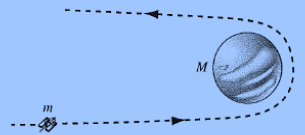Concept:-

In accordance to the law of conservation of momentum, the total momentum of the particle having mass m1 and the particle having mass m2 before the collision equals their total momentum after the collision.  The changes in momentum of the two objects have equal magnitudes and opposite signs, a necessary consequence of the law of conservation of momentum.

In an elastic collision, the final velocity v1f of the body having mass m1 is,

v1f = (m1 – m2/ m1 + m2) v1i + (2m2/ m1 + m2) v2i

and the final velocity v2f of the body having mass m2 is,

v2f = (2m1/ m1 + m2) v1i + (m2 – m1/ m1 + m2) v2i

Here, v1i is the initial velocity of the body having mass m1 and v2i is the initial velocity of the body having mass m2.

When, m1 >> m2, then,

v1f = v1i and v2f ≈ 2v1i - v2i

Solution:-

Since the mass of Jupiter M (m1 = M) is very much greater than the mass of the spacecraft m (m2 = m), therefore the speed of the spacecraft v2f relative to the Sun will be,

v2f ≈ 2v1i - v2i

≈ 2V – v      (Since, V = v1i and v =v2i)

Here, speed of the Jupiter relative to the Sun is V (=v1i) and speed of the spacecraft relative to the Sun is v (=v2i).

To obtain the speed v2f of the spacecraft relative to the Sun, after the “slingshot” encounter, substitute 13 km/s for speed of the Jupiter relative to the Sun V and -12 km/s for speed of the spacecraft relative to the Sun v in the equation v2f = 2V – v,

v2f = 2V – v

= 2(13 km/s) – (-12 m/s)

= (26 km/s) + (12 km/s)

= 38 km/s

From the above observation we conclude that, the speed v2f of the spacecraft relative to the Sun, after the “slingshot” encounter would be 38 km/s.

_______________________________________________________

Probelm 6:-

It is well known that bullets and other missiles fired at Super man simply bounce off his chest as shown in below figure. Suppose that a gangster sprays Superman’s chest with 3.0-g bullets at the rate of 100 bullets/min, the speed of each bullet being 500 m/s. Suppose too that the bullets rebound straight back with no loss in speed. Find the average force exerted by the stream of bullets on Superman’s chest.Concept:-

The impulse of the net force J acting on a particle during a given time interval is equal to the change in momentum Δp of the particle during that interval.

So, J = Δp

= pf - pi

Here pf is the final momentum and pi is the initial momentum of the body.

Impulse of a force J is defined as,

J = Fav t

Here Fav is the average force and t is the impact time.

J = Fav t

So, Fav = J/t

Solution:-

The change in momentum Δp of one bullet will be,

Δp = pf - pi

= mv- (-mv)

= 2mv

To obtain the change in momentum Δp of one bullet, substitute 3.0 g for mass m and 500 m/s for v in the equation Δp = 2mv,

Δp = 2mv

= 2(3.0 g) (500 m/s)

= 2(3.0 g×10-3 kg/1 g) (500 m/s)

= 3.0 kg.m/s

The average force Fav is equal to the total impulse J in one minute divided by one minute.

So, Fav = 100(J)/t

= 100(Δp)/t

To find out the average force Fav exerted by the stream of bullets on Superman’s chest, substitute 3.0 kg.m/s for Δp and 60 s for t in the equation Fav = 100(Δp)/t,

Fav =100(Δp)/t

=100(3.0 kg.m/s)/(60 s)

=(5.0 kg.m/s2) (1 N/1 kg.m/s2)

= 5.0 N

Thus from the above observation we conclude that, the average force Fav exerted by the stream of bullets on Superman’s chest would be 5.0 N.

______________________________________________________________

Problem 7:-

The two spheres on the right of below figure are slightly separated and initially at rest; the left sphere is incident with speed v0. Assuming head-on elastic collision, (a) if m ≥ M, show that there are two collisions and find all final velocities; (b) if m<M, show that there are three collisions and all final velocities.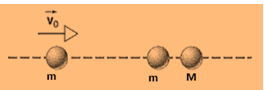Concept:-

In accordance to the law of conservation of momentum, the total momentum of the particle having mass m1 and the particle having mass m2 before the collision equals their total momentum after the collision. The changes in momentum of the two objects have equal magnitudes and opposite signs, a necessary consequence of the law of conservation of momentum.

In an elastic collision, the final velocity v1f of the body having mass m1 is,

v1f = (m1 – m2/ m1 + m2) v1i + (2m2/ m1 + m2) v2i

and the final velocity v2f of the body having mass m2 is,

v2f = (2m1/ m1 + m2) v1i + (m2 – m1/ m1 + m2) v2i

Here, v1i is the initial velocity of the body having mass m1 and v2i is the initial velocity of the body having mass m2.

Solution:-

As the target particle second cart (m2) is at rest, thus, v2i = 0.

Thus, v1f = (m1 – m2/ m1 + m2) v1i and v2f = (2m1/ m1 + m2) v1i

The two spheres in the below figure are slightly separated and initially at rest; the left sphere is incident with speed v0. As head-on collision occurs so there will always be at least two collisions. Consider the balls are a,b, and c from left to right.

After the first collision between a and b one has, the velocity of b will be,

vb,1= v0

and

the velocity of a will be,

va,1= 0

After the first collision between b and c, the velocity of c will be,

vc,1=2mv0/m+M

and

the velocity of b will be,

vb,2=(m-M)v0/(m+M)

(a) If m ≥ M then the ball b continue to move to right (or stops) and there are no more collisions.

(b) If m < M then ball b bounces back and strikes ball a which was at rest.

Then,

va,2 = (m-M)v0/(m+M)

and

vb,3= 0

__________________________________________________________________

Problem 8:-

Two cars A and B slide on an icy road as they attempt to stop at a traffic light. The mass of A is 1100 kg and the mass of B is 1400 kg. The coefficient of kinetic friction between the locked wheels of both cars and the road is 0.130. Car A succeeds in coming to rest at the light, but car B cannot stop and rear-ends car A. After the collision, A comes to rest 8.20 m ahead of the impact point and B 6.10 m ahead: as shown in the below figure. Both drivers had their brakes locked throughout the incident. (a) From the distances each car moved after the collision, find the speed of each car immediately after impact. (b) Use conservation  of momentum to find the speed at which car B struck car A. On what grounds can the use of momentum conservation be criticized here?Concept:-

Acceleration a due to friction is defined as,

a =µkg

Here µk is the coefficient of kinetic friction and g is the free fall acceleration.

Time t is equal to the speed v of the object divided by the acceleration a of the object.

t = v/a

In accordance to equation of motion, the distance d traveled by the object is equal to,

d = ut+1/2at2

Here u is the initial velocity, t is the time and a is the acceleration.

As the initial velocity u=0, thus the equation d = ut+1/2at2 will become,

d = ut+1/2at2

= (0)t+1/2at2

= 1/2at2

Solution:-

(a) For an object with initial speed v and deceleration –a which travels a distance x before stopping.

So the time t to stop will be,

t=v/a

The average speed while stopping is v/2.

The distance x will be,

x=1/2at2

To obtain the speed v, substitute v/a for t in the equation d=1/2at2, we get,

x=1/2at2

= 1/2a(v/a)2

2x=v2/a

Or,v=√2ax

To find out speed in terms of coefficient friction, Substitute µkg for a in the equation v=√2ax,

v=√2ax

=√2 (µkg) x

=√2µkg x

To obtain the speed of car A after the collision, substitute 0.130 for µk, 9.81m/s2 for g and 8.20 m for x in the equation v=√2µkg x, we get,

v=√2µkg x

=√2(0.130)(9.81m/s2)(8.20 m)

=4.57 m/s

To obtain the speed of car B after the collision, substitute 0.130 for µk, 9.81 m/s2 for g and 6.10 m for x in the equation v=√2µkg x, we get,

v=√2µkg x

=√2(0.130)(9.81m/s2)(6.10 m)

=3.94 m/s

From the above observation we conclude that, the speed of car A after the collision will be 4.57 m/s while the speed of car B will be 3.94 m/s.

(b) If v0 is the at which car B struck car A, then in accordance to law of conservation of linear momentum,

mB v0 = mAvA+ mBvB

So, v0 = mAvA+ mBvB/ mB

Here mA is the mass of car A, mB is the mass of car B, vA is the speed of car A and vB is the speed of car B.

To obtain the speed v0 at which car B struck car A, substitute 1100 kg for mA, 4.57 m/s for vA, 1400 kg for mB and 3.94 m/s for vB in the equation  v0 = mAvA+ mBvB/ mB,

v0 = mAvA+ mBvB/ mB

=[(1100 kg)(4.57 m/s)+(1400 kg)(3.94 m/s)]/(1400 kg)

=7.53 m/s

From the above observation we conclude that, the speed v0 at which car B struck car A would be 7.53 m/s.

_________________________________________________________________

Problem 9:-

A karate expert breakes a pine board, 2.2 cm thick, with a hand chop. Strobe photography shows that the hand, whose mass may be taken as 540 g, strikes the top of the board with a speed of 9.5 m/s and comes to rest 2.8 cm below this level. (a) What is the time duration of the shop (assuming a constant force)? (b) What average force is applied?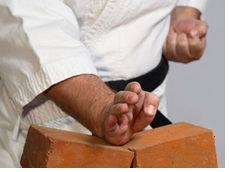Concept:-

Average speed vav is equal to the total distance y travelled by the body divided by total time t taken by the body to travel that distance.

So, vav = y/t

Thus the time t will be,

t = y/ vav

Momentum of a body (p) is defined as,

p = mv

Here, m is the mass of the body and v is the velocity of the body.

The impulse of the net force J acting on a particle during a given time interval is equal to the change in momentum Δp of the particle during that interval.

So, J = Δp

= mΔv    (Since, Δp = mΔv)

Impulse of a force J is defined as,

J = Fav t

Here Fav is the average force and t is the impact time.

J = Fav t

So, Fav = J/t

Solution:-

(a) The average speed vav during the time the hand is in contact with the board is half of the initial speed.

Thus, vav = (9.5 m/s) /2 = 4.8 m/s

To obtain the time duration t of the chop, substitute 2.8 cm for y and 4.8 m/s for vav in the equation t = y/ vav,

t = y/ vav

= (2.8 cm)/(4.8 m/s)

= (2.8 cm×10-2 m/1 cm)/(4.8 m/s)

= 5.8×10-3 s

= (5.8×10-3 s) (1 ms/10-3 s)

= 5.8 ms

Therefore the time duration t of the chop will be 5.8 ms.

(b) To find out the applied average force Fav, first we have to find out the impulse of force J.

The impulse given to the board is the same as the magnitude in the change in momentum of the hand.

To obtain impulse J given to the board, substitute 540 g for m and 9.5 m/s for Δv  in the equation J = mΔv,

J = mΔv

= (540 g) (9.5 m/s)

= (540 g× 1 kg/103g) (9.5 m/s)

= (5.1 kg.m/s) (1 N/1 kg.m/s2)

= 5.1 N.s

To obtain the applied average force Fav, substitute 5.1 N.s for J and 5.8 ms for t in the equation Fav = J/t,

Fav = J/t

=(5.1 N.s) / (5.8 ms)

= (5.1 N.s) / (5.8 ms×10-3 s/1 ms)

= 880 N

From the above observation we conclude that, the applied average force Fav would be 830 N.

__________________________________________________________________________

Problem 10:-

A 195-lb man standing on a surface of negligible friction kicks forward a 0.158-lb stone lying at his feet so that it acquires a speed of 12.7 ft/s. What velocity does the man acquires as a result?

Concept:-

Law of conservation of linear momentum states that, in an isolated system (no external force), the algebraic sum of momenta of bodies, along any straight line, remains constant and is not changed due to their mutual action and reaction on each other. Therefore total momentum of the system before collision is equal to the total momentum of the system after collision.

The momentum of particle p is equal to the mass of particle m times the velocity of particle v.

So, p = mv    …… (1)

Solution:-

Since both the body is initially rest, therefore the velocities of both the bodies are zero resulting the initial momentum of the system will be zero.

So, initial momentum of the system = 0

Let us consider mm is the mass of the man and vm is the velocity of the man when the man kicks the stone forward.

So using equation (1), the momentum of the man (pm) when he kicks the stone forward will be,

pm= mmvm          …… (2)

Let us consider ms is the mass of the stone and vs is the velocity of the stone after kicked by the man.

So again using equation (1), the momentum of the stone (p2) after kicked by the man

will be,

ps= msvs            …… (3)

Final momentum of the system = pm + ps

=  mmvm + msvs     …… (4)

Conservation of linear momentum states that, the algebraic sum of momenta of bodies, along any straight line, remains constant and is not changed due to their mutual action and reaction on each other. Therefore total momentum of the system before collision is equal to the total momentum of the system after collision.

So,

pm + ps = 0

So, mmvm + msvs = 0

mmvm = -msvs

vm = -msvs/mm          …… (5)

To obtain the velocity of the man, substitute 0.158-lb for mass of the stone ms, 12.7 ft/s for speed of the stone vs and 195-lb for mass of the man mm in the equation vm = -msvs/mm.

So, vm = -msvs/mm

= - (0.158-lb) (12.7 ft/s)/(195-lb)

= -1.0×10-2 ft/s        …… (6)

From equation (5) we observed that, the velocity of the man will be -1.0×10-2 ft/s.

Related Resources:-

You might like to refer Conservation of Momentum.

For getting an idea of the type of questions asked, refer the  Previous Year Question Papers.

```### Course Features

• 101 Video Lectures
• Revision Notes
• Previous Year Papers
• Mind Map
• Study Planner
• NCERT Solutions
• Discussion Forum
• Test paper with Video Solution